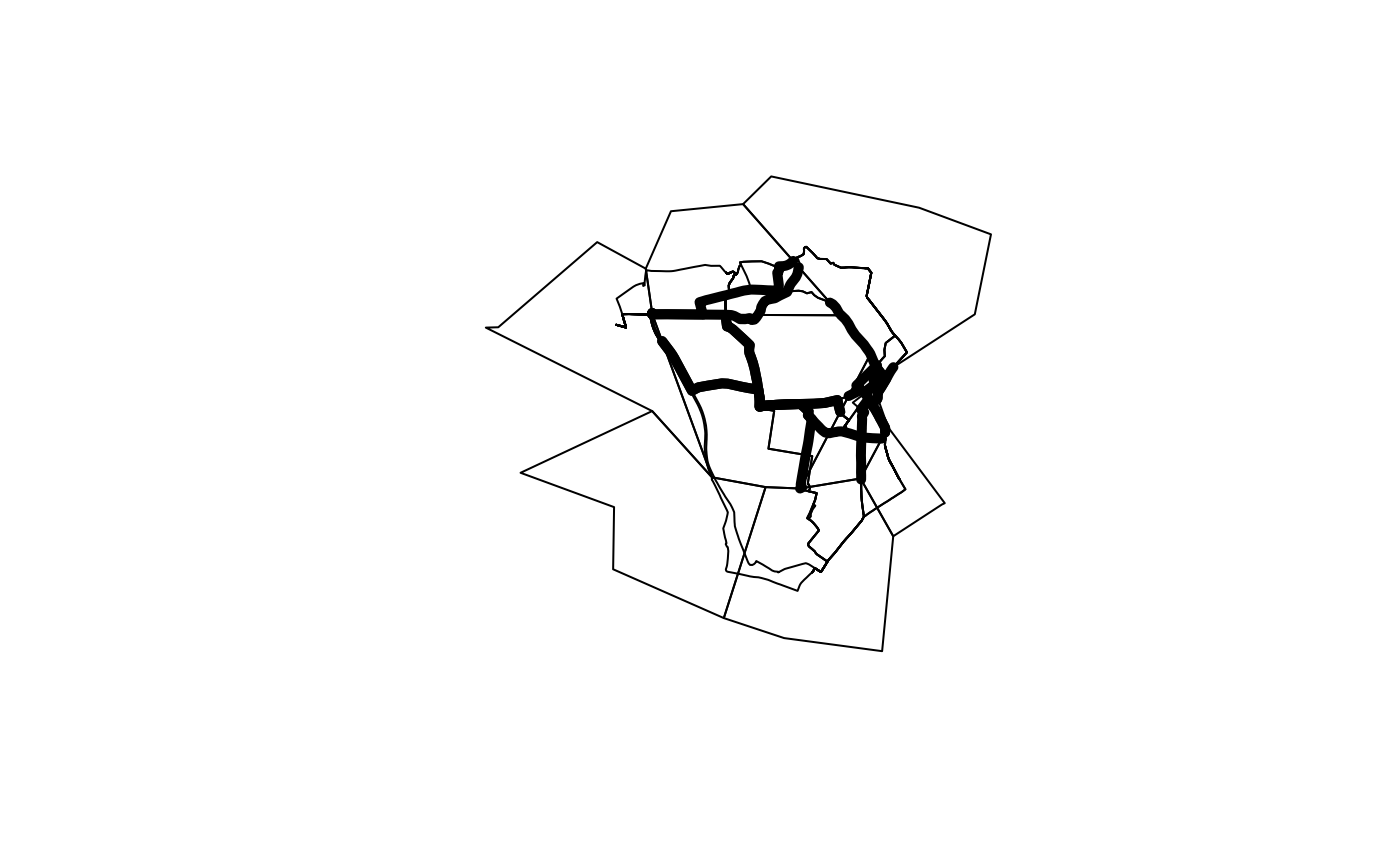Takes lines and removes the start and end point, to a distance determined by the nearest `buff` polygon border.

## Usage

``toptail_buff(l, buff, ...)``

## Arguments

l

An `sf` object representing lines

buff

An `sf` object with POLYGON geometry to buffer the linestring.

...

Arguments passed to `sf::st_buffer()`

Other lines: `angle_diff()`, `geo_toptail()`, `is_linepoint()`, `line2df()`, `line2points()`, `line_bearing()`, `line_breakup()`, `line_midpoint()`, `line_segment()`, `line_via()`, `mats2line()`, `n_vertices()`, `onewaygeo()`, `points2line()`

## Examples

``````l <- routes_fast_sf
buff <- zones_sf
r_toptail <- toptail_buff(l, buff)
nrow(l)
#>  49
nrow(r_toptail)
#>  8
plot(zones_sf\$geometry)